# 题目描述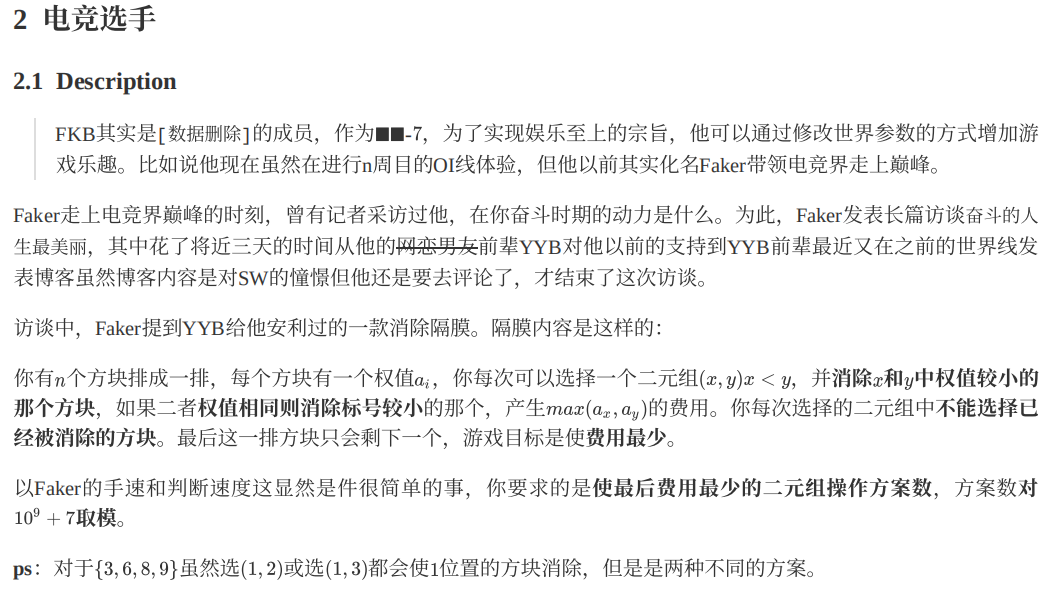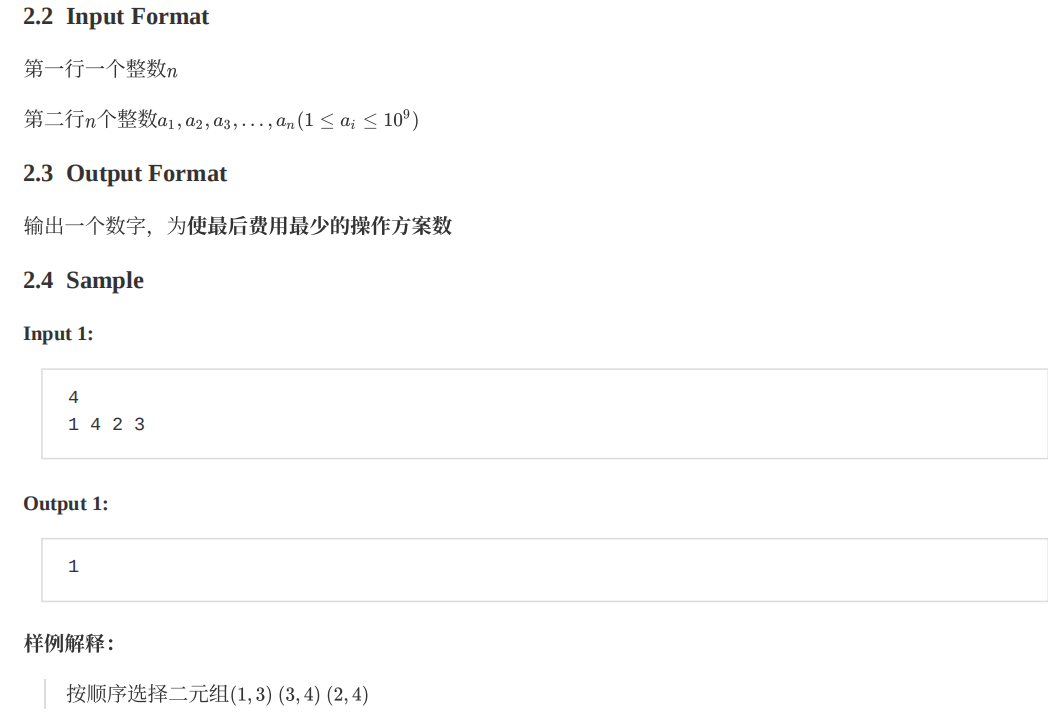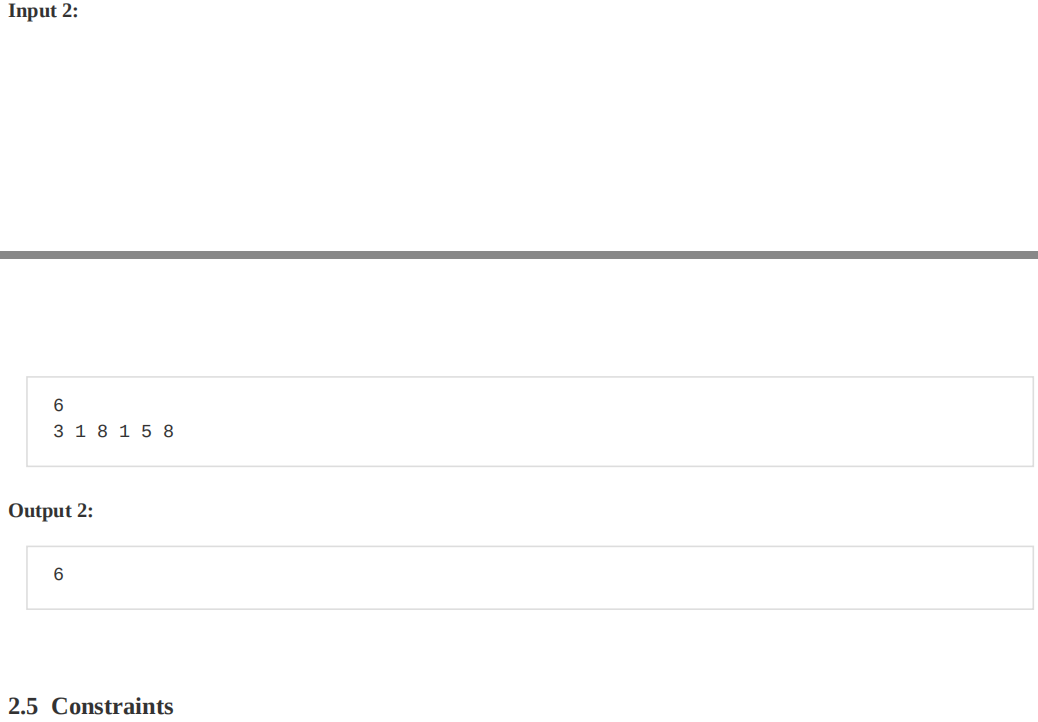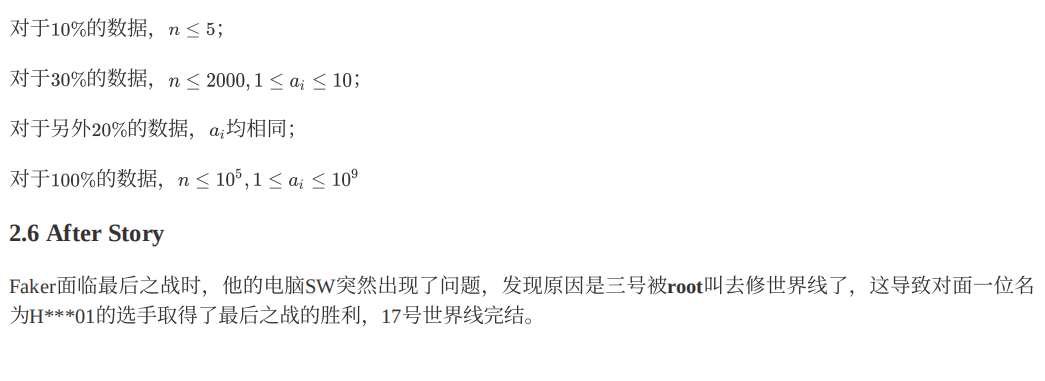# 心路历程

f[n]=f[n-1]*C(n,2)

# 规律

$ans=\prod{每段的f}*\prod{C_{从1到当前段末尾长度}^{当前段长度-1}(当前段不是第一段)}$

a=1 1 2 2 2 3 4 4

# 证明

$f=1$
$f[n]=f[n-1]*C_n^2 (n>1)$

# 关于规律的证明

$ans=\prod{f[每段长度]}*\prod{C_s^{n-1}(第≥2段)}$

f已经算过了，接下来就是证明
$\sum_{i=s-n-1}^{s-2}{C_i^{s-n-1}*(s-i-1)}=C_s^{n-1}$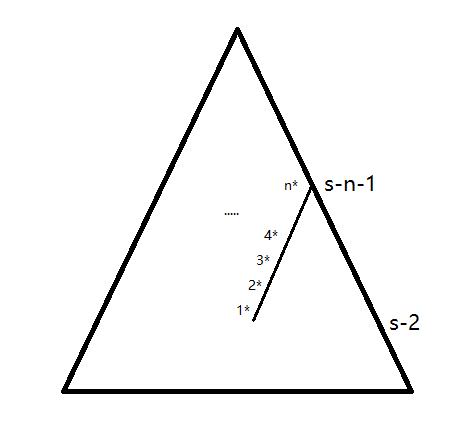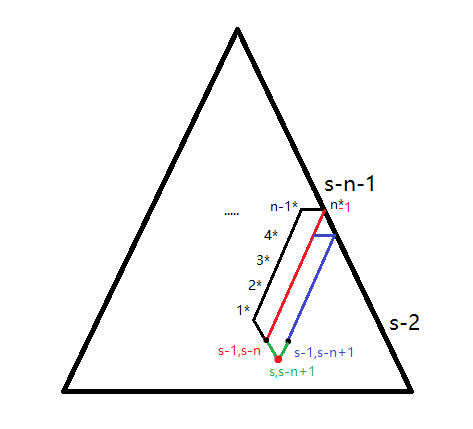$\sum_{i=s-n-1}^{s-2}{C_i^{s-n-1}*(s-i-1)}=C_s^{n-1}$

（因为之前写了一遍被csdn吞了，所以不想再详写）

# code

#include <algorithm>
#include <iostream>
#include <cstdlib>
#include <cstdio>
#define fo(a,b,c) for (a=b; a<=c; a++)
#define fd(a,b,c) for (a=b; a>=c; a--)
#define mod 1000000007
#define Mod 1000000005
using namespace std;

long long f;
long long jc;
long long Jc;
int a;
int n,i,j,k,l;
long long sum,ans;
bool bz;

long long qpower(long long a,int b)
{
long long ans=1;

a%=mod;
while (b)
{
if (b&1) ans=ans*a%mod;
b>>=1;
a=a*a%mod;
}

return ans;
}

long long C(int x,int y)
{
return jc[x]*Jc[y]%mod*Jc[x-y]%mod;
}

long long work(int sum,int num)
{
int i;
long long ans=(sum-1)*C(sum-1,sum-num)%mod;

fo(i,sum-num-1,sum-2)
ans=(ans-C(i,sum-num-1)*i)%mod;

return ans;
}

int main()
{
jc=1;
Jc=1;

sum=0;
f=1;

scanf("%d",&n);
fo(i,1,n)
{
scanf("%d",&a[i]);

jc[i]=jc[i-1]*i%mod;
Jc[i]=qpower(jc[i],Mod);

sum=(sum+i)%mod;
f[i+1]=(f[i]*sum)%mod;
}

sort(a+1,a+n+1);

sum=0;ans=1;
fo(i,1,n)
{
if (a[i-1]!=a[i])
sum=1;
else
++sum;

if (i==n || a[i]!=a[i+1])
{
ans=ans*f[sum]%mod;

if (bz)
//			ans=ans*work(i,sum)%mod;
ans=ans*C(i,sum-1)%mod;
else
bz=1;
}
}

ans+=(ans<0)?mod:0;
printf("%lld\n",ans);

return 0;
}